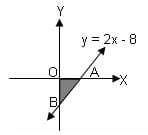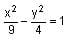CAT  >  Practice Questions Level 1: Coordinate Geometry

# Practice Questions Level 1: Coordinate Geometry - Notes | Study Level-wise Practice Questions for CAT Preparation - CAT

 1 Crore+ students have signed up on EduRev. Have you?

This EduRev document offers 15 Multiple Choice Questions (MCQs) from the topic Coordinate Geometry (Level - 1). These questions are of Level - 1 difficulty and will assist you in the preparation of CAT & other MBA exams. You can practice/attempt these CAT Multiple Choice Questions (MCQs) and check the explanations for a better understanding of the topic.

Question for Practice Questions Level 1: Coordinate Geometry
Try yourself:The image of the point (α, β) in the line x + y = 0 is

Question for Practice Questions Level 1: Coordinate Geometry
Try yourself:In the diagram, the line with equation y = 2x - 8 crosses x-axis at A and y-axis at B. The area of ΔAOB isQuestion for Practice Questions Level 1: Coordinate Geometry
Try yourself:If x-form (x, y) = (x2 + x, y2 - y) and y-form (x, y) = (x2 - 11 , y2) are two functions defined on a plane, then find the distance between the points x-form (-2, 5) and y-form (5, -2)

Question for Practice Questions Level 1: Coordinate Geometry
Try yourself:Find the equation of the straight line passing through the point (-2, -3) and perpendicular to the line passing through (-2, 3) and (-5, -6).

Question for Practice Questions Level 1: Coordinate Geometry
Try yourself:The lines a1x + b1y + c1 = 0 and a2x + b2y + c2 = 0 are perpendicular to each other if

Question for Practice Questions Level 1: Coordinate Geometry
Try yourself:Find the coordinates of the point which divides P1(1, -4) and P2(4, 2) externally in the ratio 1 : 4.

Question for Practice Questions Level 1: Coordinate Geometry
Try yourself:The vertices of a triangle are at (0, 0), (8, 0) and (0, 14). The orthocentre of the triangle is at

Question for Practice Questions Level 1: Coordinate Geometry
Try yourself:If A (a, 0) and B (3a, 0) are the vertices of an equilateral triangle ABC, then what are the coordinates of C?

Question for Practice Questions Level 1: Coordinate Geometry
Try yourself:The equation of the straight line which passes through the point (3, 4) and makes an intercept on the y-axis twice as long as that on the x-axis is

Question for Practice Questions Level 1: Coordinate Geometry
Try yourself:The value of k for which the circles x2 + y2 - 3x + ky - 5 = 0 and 4x2 + 4y2 - 12x - y - 9 = 0 become concentric is

Question for Practice Questions Level 1: Coordinate Geometry
Try yourself:What is the equation of a straight line which passes through the point of intersection of the straight lines x – 3y + 1 = 0 and 2x + 5y – 9 = 0 and has infinite slope?

Question for Practice Questions Level 1: Coordinate Geometry
Try yourself:The curvepasses through the point

Question for Practice Questions Level 1: Coordinate Geometry
Try yourself:A triangle has 12 units base on the line 3x + 7y = 12. If the third vertex is at point (3, - 5), find the area of the triangle.

Question for Practice Questions Level 1: Coordinate Geometry
Try yourself:The area of the circle x2 + y2 - 6x - 8y - 24 = 0 is

Question for Practice Questions Level 1: Coordinate Geometry
Try yourself:If u = a1x + b1y + c1 = 0, v = a2x + b2y + c2 and a1/a2 = b1/b2 = c1/c2 , then locus of the equation u + λv = 0, λ ∈ R is

The document Practice Questions Level 1: Coordinate Geometry - Notes | Study Level-wise Practice Questions for CAT Preparation - CAT is a part of the CAT Course Level-wise Practice Questions for CAT Preparation.
All you need of CAT at this link: CAT

## Level-wise Practice Questions for CAT Preparation

277 docs
 Use Code STAYHOME200 and get INR 200 additional OFF

## Level-wise Practice Questions for CAT Preparation

277 docs

### How to Prepare for CAT

Read our guide to prepare for CAT which is created by Toppers & the best Teachers

Track your progress, build streaks, highlight & save important lessons and more!

,

,

,

,

,

,

,

,

,

,

,

,

,

,

,

,

,

,

,

,

,

;